# Six Sigma DMAIC Process - Analyze Phase - Data Door Analysis

Let us learn about a few Representation Tools that help us in analyzing the data and also representing them appropriately.

Process variation can be classified as Variation for a period of Time and Variation Over Time. Variation for a period of time can be defined for discrete and continuous data types as below:
• Discrete Data: Bar Diagram, Pie Chart, Pareto Chart
• Continuous Data: Histogram, Box Plot
Variation Over Time can be defined for discrete and continuous data types as:
• Discrete Data: Run Charts, Control Chart
• Continuous Data: Run Chart, Control Chart
Bar Diagram:
A bar diagram is a graphical representation of attribute data. It is constructed by placing the attribute values on the horizontal axis of a graph and the counts on the vertical axis.Six Sigma Bar Diagram

Pie Chart:
A pie chart is a graphical representation of attribute data. The “pieces” represent proportions of count categories in the overall situation. Pie charts show the relationship among quantities by dividing the whole pie (100%) into wedges or smaller percentages.Six Sigma Pie Chart

Cause and Effect Diagram / Fish Bone Diagram / Ishikawa Diagram:
This is a visual tool used to brainstorm the probable causes for a particular effect to occur. Effect or the problem is analogously captured as the head of the fish and thus the name. The causes for this effect or problem is generated through team brainstorming and are captured along the bones of the fish. The causes generated in the brainstorming exercises by the team will depend on how closely the team is related to the problem. Typically the causes are captured under predetermined categories such as 6M’s or 5M’s and a P as given below:
• Machine: This category groups root causes related to tools used to execute the process.
• Material: This category groups root causes related to information and forms needed to execute the process.
• Nature: This category groups root causes related to our work environment, market conditions, and regulatory issues.
• Measure: This category groups root causes related to the process measurement.
• Method: This category groups root causes related to procedures, hand-offs, input-output issues.
• People: This category groups root causes related people and organizations.
Below is an example of a fishbone diagram created for capturing the root causes of High Turn Around Time (TAT).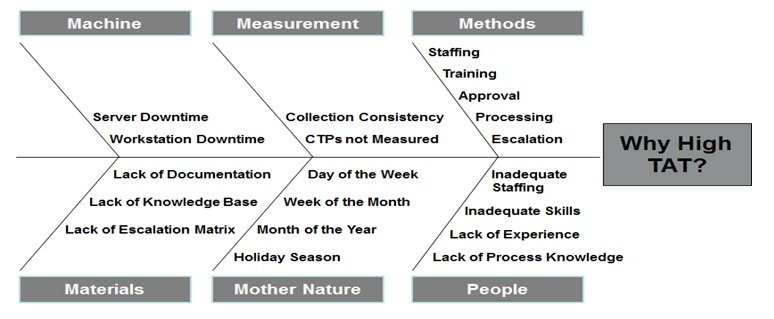Six Sigma Cause and Effect Diagram / Fish Bone Diagram / Ishikawa Diagram

Pareto Chart:
A data display tool for numerical data that breaks down discrete observations into separate categories for the purpose of identifying the "vital few".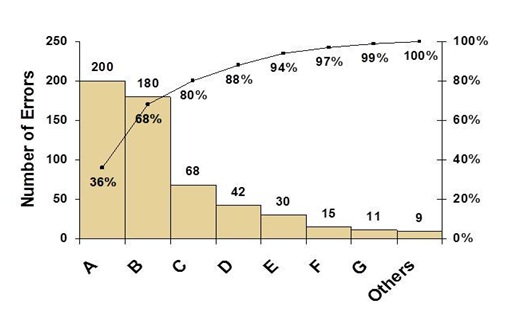Six Sigma Pareto Chart

Histogram:
A histogram is a graphical representation of numerical data. It is constructed by placing the class intervals on the horizontal axis of a graph and the frequencies on the vertical axis.Six Sigma Histogram

Box Plot:
A box plot summarizes information about the shape, dispersion, center of process data and also helps spot outliers in the data.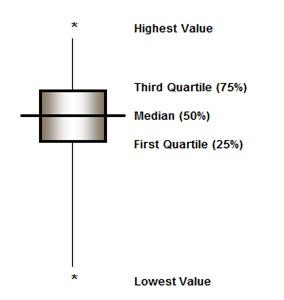Six Sigma Box Plot

The box plot can be interpreted as follows:
• Box – represents the middle 50% values of the process data.
• Median – represents the point for which 50% of the data points are above and 50% are below the line.
• Q1, Q3 – Q1 represents the point for which 25% of the data points are above and 75% are below the line; While, Q3 represents the point for which 75% of the data are above and 25% are below in the line.
• Aestrix – represents an outlier and is a point which is more than 1.5 times the inter-quartile range (Q3-Q1) in the data.
• Lines – These vertical lines represent a whisker which joins Q1 or Q3 with the farthest data-point but other than an outlier.
Example: Below is an example of a call center process where Average Handle Time (AHT) of the calls is compared between Team Leads of the process.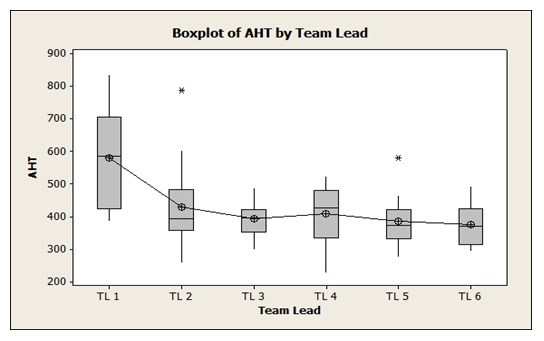Six Sigma Box Plot Example

You will observe that the variation is highest for TL1 and for the rest it is much smaller. This indicates that the associates working under TL1 need training or some other help which will reduce the variation and bring the overall AHT under control. Scatter Plot:
A scatter plot is often employed to identify potential associations between two variables, where one may be considered to be an explanatory variable (such as years of education) and another may be considered a response variable (such as annual income).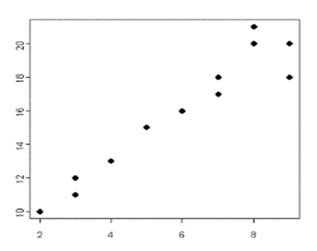Six Sigma Scatter Plot

Scatter plots are similar to line graphs in that they use horizontal and vertical axes to plot, large body of, data points. And, they have a very specific purpose too:
• They show how much one variable is affected by another variable and this relationship is called as their correlation.
• The closer the data points come when plotted to making a straight line, higher is the correlation between variables.
• If the data points make a straight line going from the origin out to high x- and y-values, then the variables are said to have a +ve correlation.
• If the line goes from a high-value on y-axis down to a high-value on x-axis, the variables have –ve correlation.
Once after identifying the factors we need to
• What is the extent of impact of the factors?
• Which one do you control?

# We guarantee that Your Free Online Training will make you pass Your Six Sigma Certification Exam!